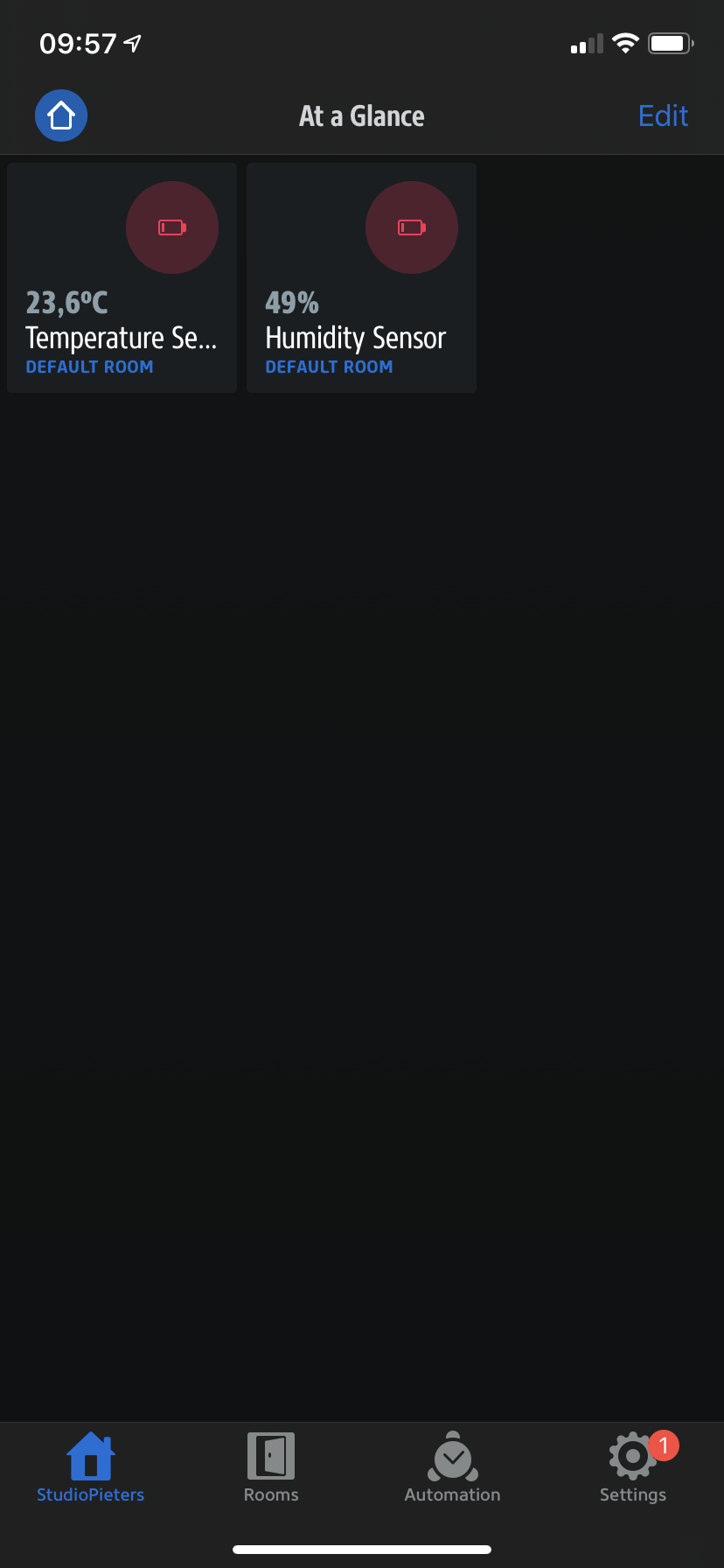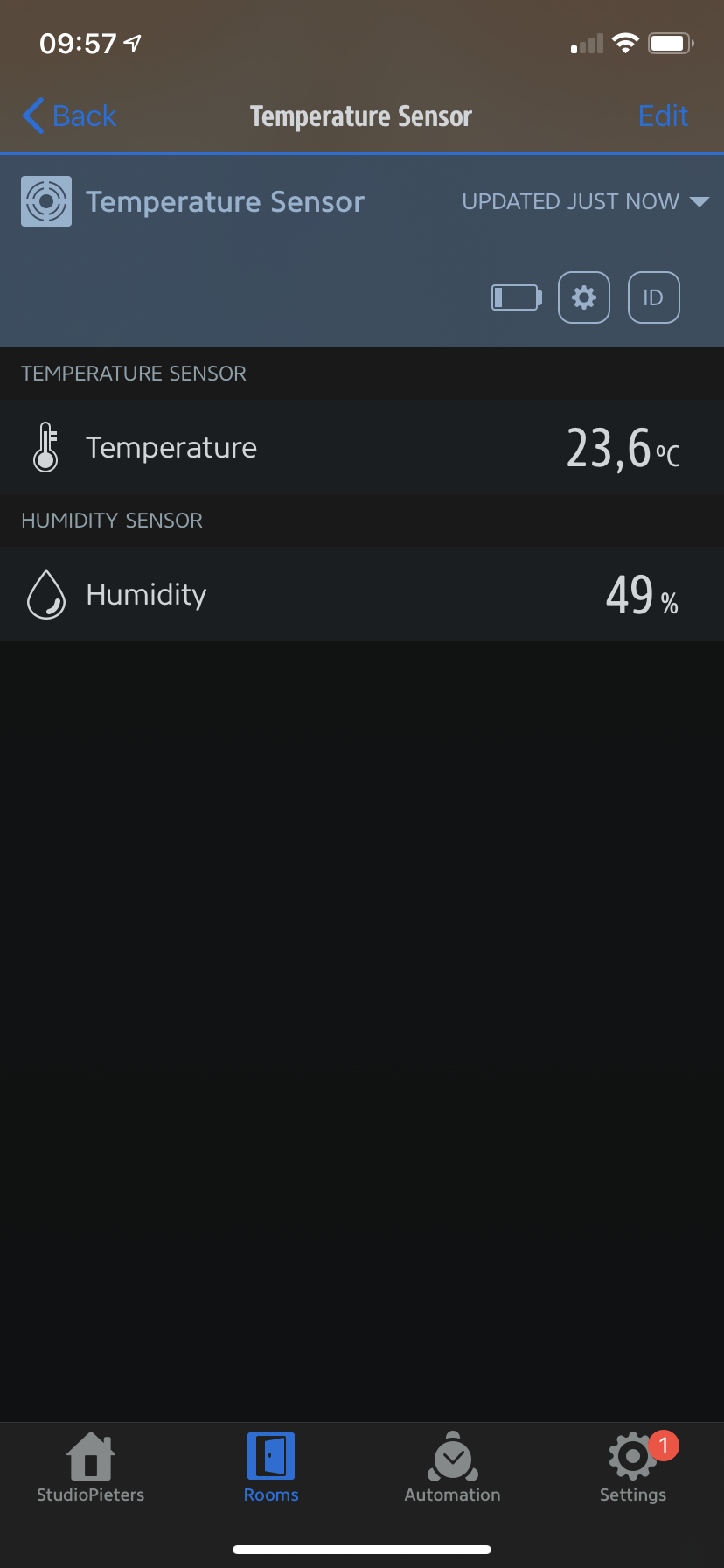# ESP8266 HomeKit – Battery Status

Slowly but surely I am looking at the extensions of the “standard” ESP HomeKit accessories, how to implement them. Now I am working on the Battery status. We need some circuit which can effectively measure voltage. Battery monitoring circuit is a traditional voltage divider circuit. I am going to measure 3.7 volt (lithium Polymer) battery.

## Voltage divider working and calculations

ESP8266 is a tiny device, it works on 3.3 volts. Since it is working on 3.3 volts its pins can source and sunk 3.3 volts only. Voltage greater than 3.3 volt may blow the pin or fry the ESP8266. In our case we want to measure 3.7 volt battery and ESP8266-12 ADC (Analog to Digital Channel) can only accept 1.0 volts.

The ADC pin has a 10-bit resolution, which means you’ll get values between 0 and 1024. The ESP8266 ADC pin input voltage range is 0 to 1V if you’re using the bare chip. However, most ESP8266 development boards come with an internal voltage divider, so the input range is 0 to 3.3V. So, in summary:

• ADC Voltage range in ESP8266 development boards: 0 to 3.3V (for example: ESP8266 12-E NodeMCU Kit, WeMos D1 Mini, …)
• ADC Voltage range in ESP8266 chip: 0 to 1V (for example: ESP-07 chip, ESP-12E chip, …)

We need to play smartly here. What we will do is divide the voltage between two resistors and measure only voltage across one resistor and remaining resistor voltage will be calculated mathematically. Typical voltage divider circuit and formula is given below.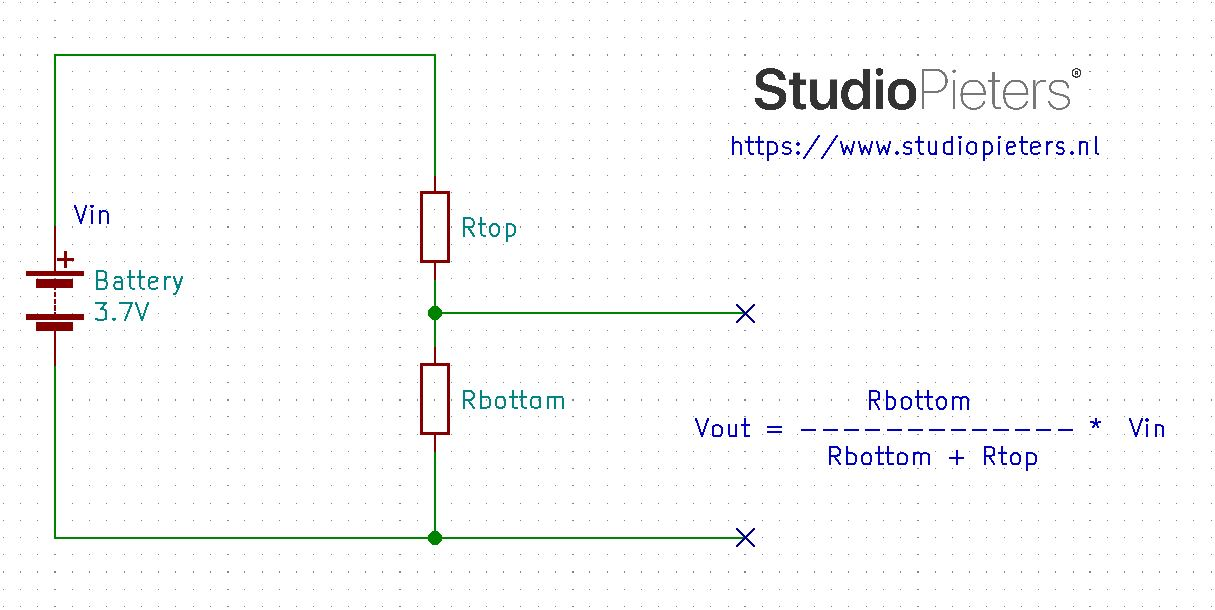Now lets calculate the values for Rtop and Rbottom. Here we need some important considerations to be taken seriously.

• Low ohm resistors can sunk much current and wires could be heated instantly. Consequently wires can melt down in seconds. So always use sufficient amount of resistors for bigger ampere hour batteries. I selected one resistor Rbottom to be 10KΩ.
• During charging battery voltage can increase to 4.2 volts

## Formula Calculations

I am going to measure voltage across Rbottom and I decided its value randomly to be 10KΩ. We know Vout can be up to maximum 3.3 volts since ESP8266 works and accepts maximum 1.0 volts at its I/O pins. Vin is 4.2 volts when the battery is charging. now we nee a formula to calculate the resistor needed.

Forumula:

 Vout = Vin Rbottom Rbottom + Rtop

When whe fill in our variables that are available, into our formula.

Forumula:

 1.0V = 4.2V 10KΩ 10KΩ + Rtop

Forumula:

 10KΩ + Rtop 42KΩ 1.0V

Forumula:

 Rtop= 42KΩ – 10KΩ

Forumula:

 Rtop = 32KΩNow if  4.2 volts are at battery side it will be divided across resistors, 1.0 volts drops at 10KΩ resistor and remaining 3.2 volts drops at 32KΩ resistor. 32KΩ ohm resistor is not available in market I am going to use the one above this rating and easily can be find from any electronics shop 33 KΩ resistor.  If battery is not charging and say supplying 3.7 volts what will be the voltage drop across resistors? Lets calculate it.

Forumula:

 Vrbottom= Vin Rbottom Rbottom + Rtop

Forumula:

 Vrbottom= 3.7V 10KΩ 32K + 10KΩ

Forumula:

 Vrbottom 37KΩ 42KΩ

Forumula:

 Vrbottom = 0.88 volts drops at rbottom

Forumula:

 Vrtop = 3.7 – 0.88 = 2.82 volts dropt at RtopIts obvious from the above discussion that the voltage across Rbottom will not exceed 1.0 Volts now. I hope it makes sense to readers about the calculations. The question now is how the 1.0 volts is converted to 3.7 volt by ESP8266 or how from 1.0 volts we can predict that at the battery side the voltage is 3.7 volts. Well a little more mathematics is involved here. Since the resistor values are fixed we can calculate the voltage ratio across the resistors with respect to the source and use it in code for actual voltage at source. How ratio is calculated is below.

 At 4.2 Volts Vbottom is 1.0 volts At 3.7 Volts Vbottom is 0.88 volts Vrbottom Ratio = 4.2V = 4.20 Vrbottom Ratio = 3.7V = 4.20 1.0V 0.88V

Two cases are given above when source is at 4.2 volt and when source is at 3.7 volt in both the cases the ratio comes out to be constant value. This ratio is utilized in code for predicting the actual battery voltage. Ratio is multiplied with voltage at Rbottom for actual voltage value. The circuit diagram is given below. I am using ADC channel of ESP8266  to measure the battery voltage. Both the battery and ESP8266 power must be grounded together in order to complete the circuit. Its a most common mistake will measuring the voltage that both grounds are not grounded together. If the ESP8266 ground is not connected with the battery ground the ADC pin will become a floating pin and it starts reading floating values.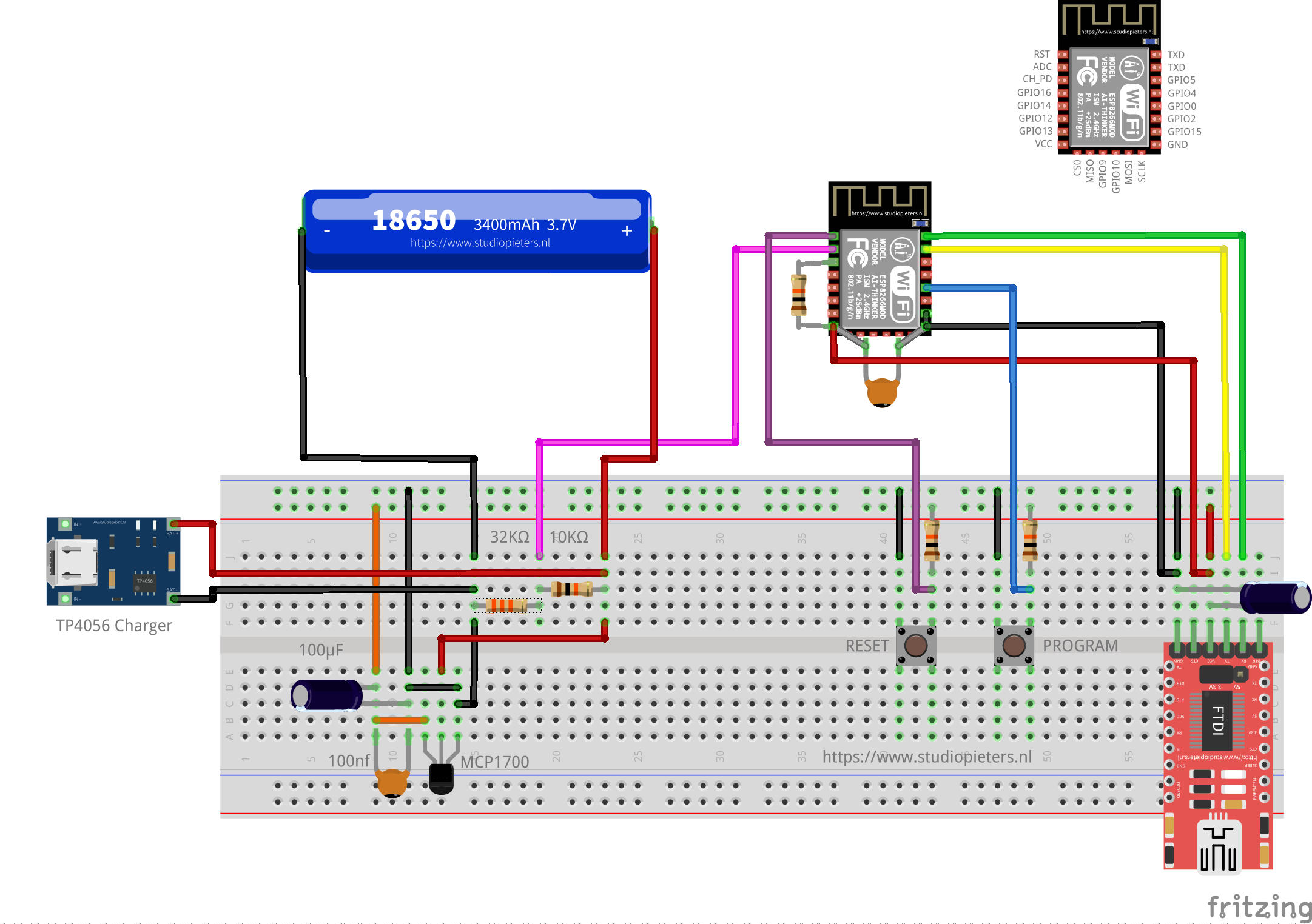After you completed the circuit its time to move on to code. Code is written in C. the most important part is where the measurement takes place.

```int low_battery_value;
float battery_value;

homekit_characteristic_t status_low_battery = HOMEKIT_CHARACTERISTIC_(STATUS_LOW_BATTERY, 0);

while (1) {
if (1.0 / 1024 * battery_value * 4.2 < 3.900) {
printf ("Battery value is low.\n");
low_battery_value = 1;
}
else{
printf ("Battery value is not low.\n");
}
homekit_characteristic_notify(&status_low_battery, HOMEKIT_UINT8(low_battery_value));
}
}
void battery_low_init() {
}

&status_low_battery,

battery_low_init();
```

Status when the battery is not low in the HomeKit App.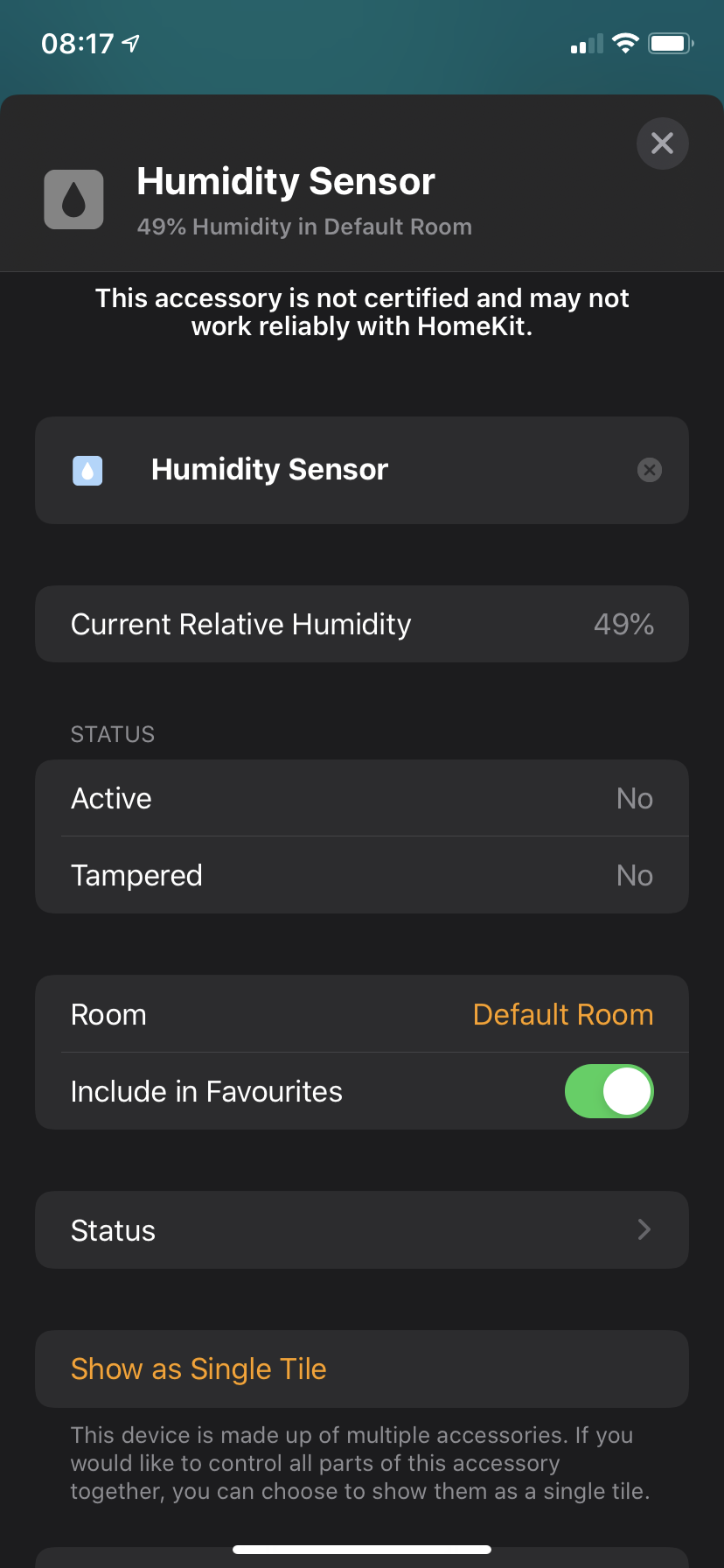status when the battery is not low in the EVE App.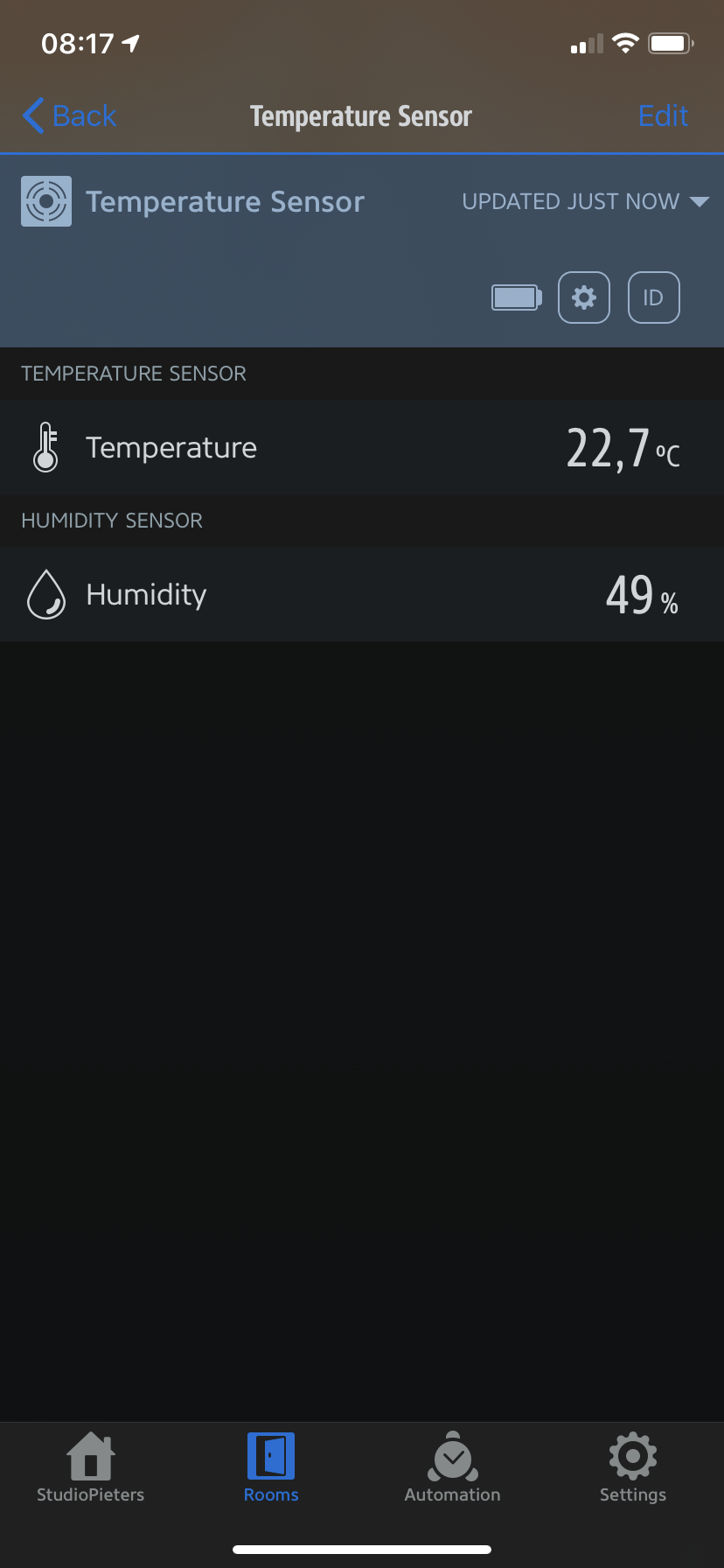Status when the battery is low in the HomeKit App. The Measurements turn red. When we click on the sensor we see that it says Low Battery. Also in the settings of the sensor you will see Battery Low.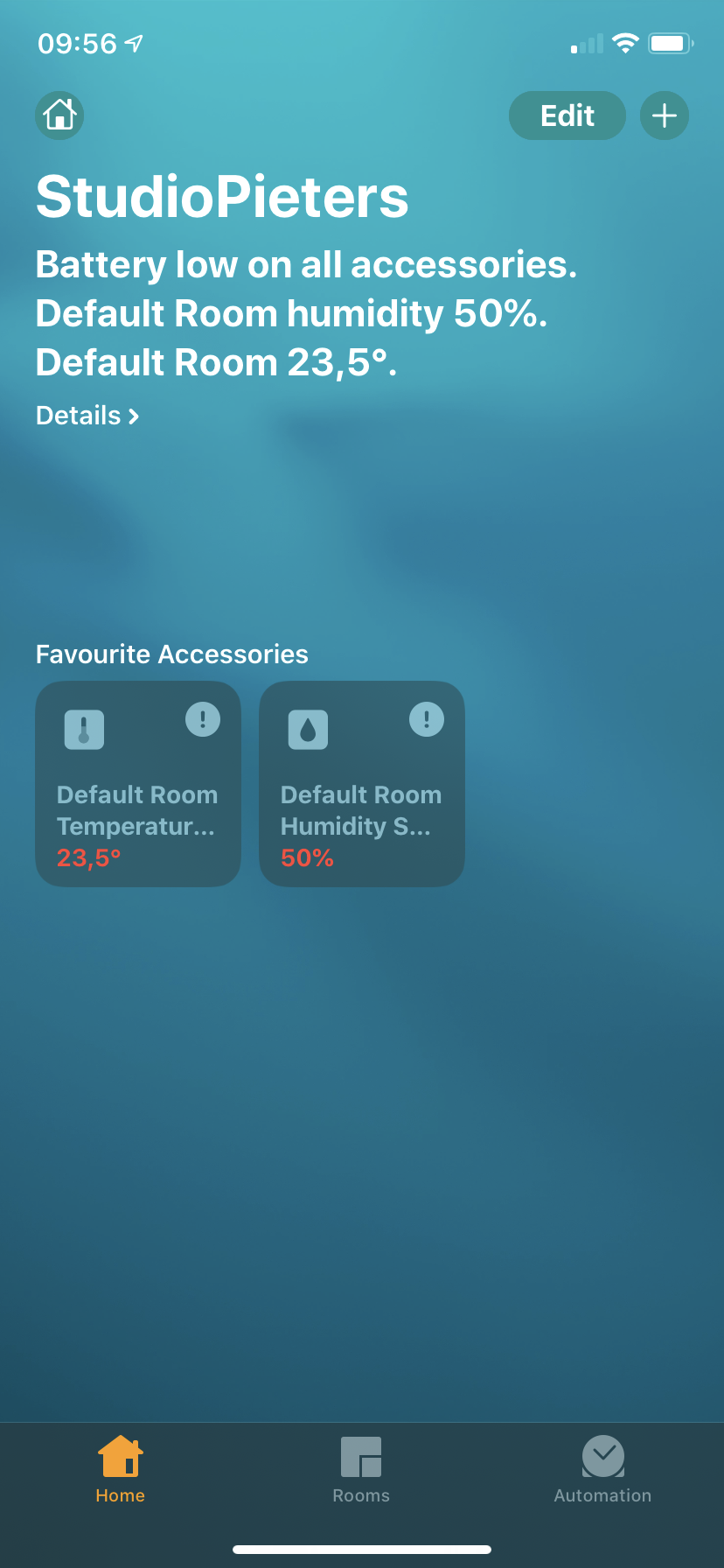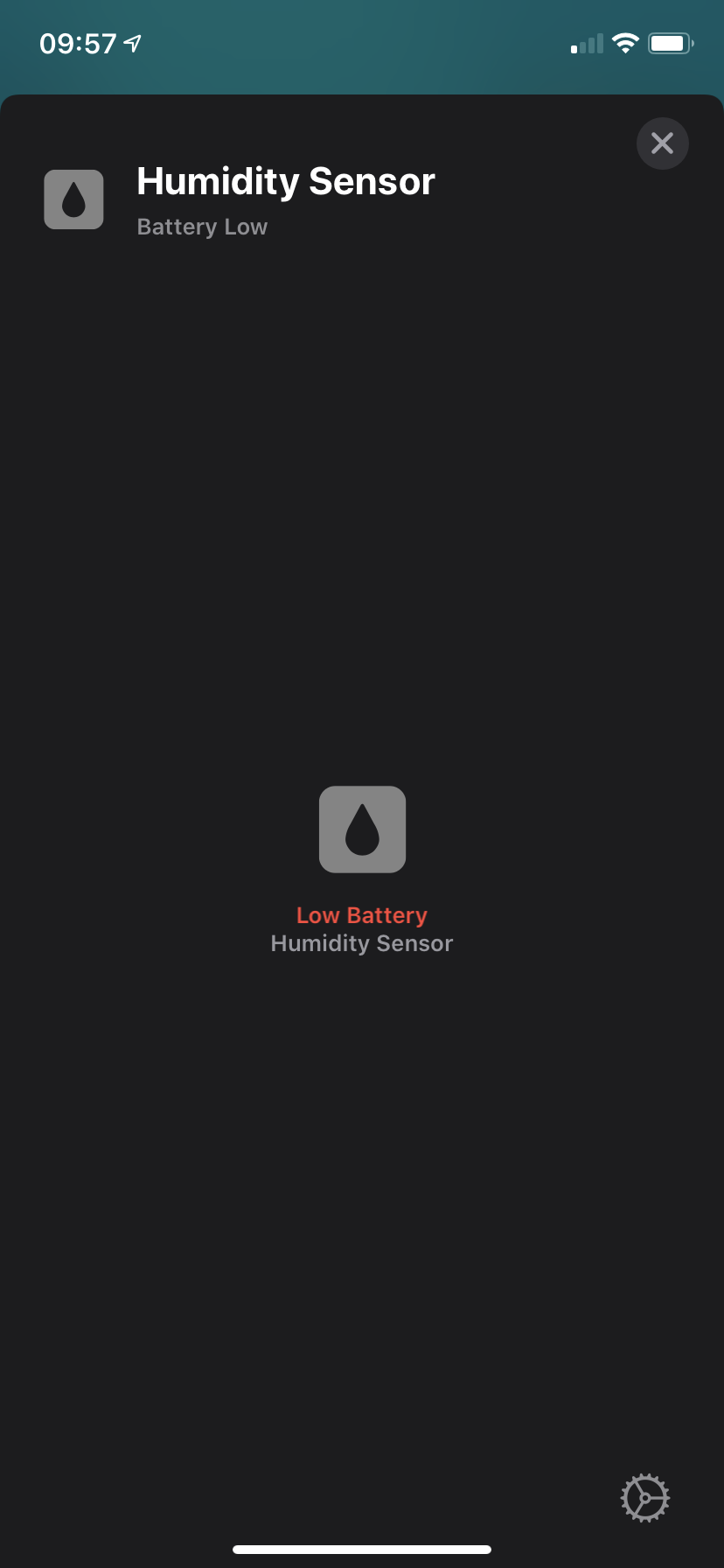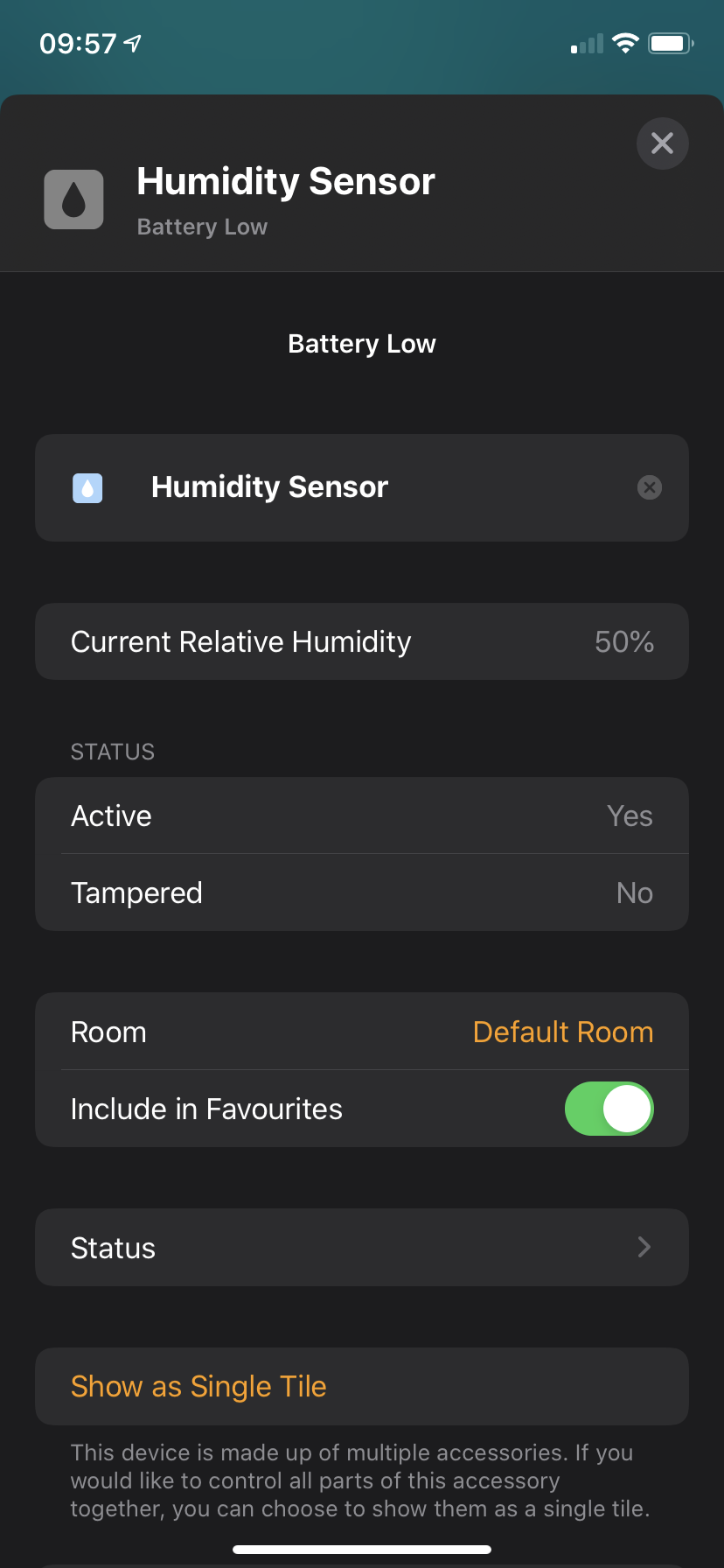Status when the battery is low in the EVE App. You will see that there will appear a Low battery icon in the sensor tile. Also in the settings of the sensor you will see Battery Low icon.